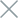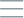• Home
• Tags
• Series
•# String Search Algorithm in C++ - Explanation, Example and Code

The string search algorithm is used to find occurrences of a specific pattern (substring) within a larger text (string). This algorithm plays a crucial role in text processing, searching, and manipulation tasks.

## How It Works

1. Start with a text (string) and a pattern (substring) to search for.
2. Iterate through the text one character at a time.
3. For each character in the text, compare it with the first character of the pattern.
4. If there is a match, check if the subsequent characters also match the pattern.
5. If the pattern is completely matched, record the starting position of the match.
6. Continue searching for the pattern in the text.

## Example

Consider a text: "ababcababcabcabc" And a pattern: "abc"

1. Start at position 0. Compare "a" with the first character "a" in the pattern.
2. Match found, move to the next characters: "b" with "b", and "a" with "c".
3. Continue matching: "b" with "a", "a" with "b", and "b" with "c".
4. Match failed at position 2.
5. Start again at position 3. Compare "a" with the first character "a" in the pattern.
6. Successful match: "a" with "a", "b" with "b", and "c" with "c".
7. Record position 3.

The pattern "abc" is found at positions 0, 6, and 9.

## Example Code in C++

``````#include <iostream>
#include <string>
#include <vector>

std::vector<int> stringSearch(const std::string& text, const std::string& pattern) {
std::vector<int> positions;

for (int i = 0; i <= text.length() - pattern.length(); ++i) {
int j = 0;
while (j < pattern.length() && text[i + j] == pattern[j]) {
++j;
}
if (j == pattern.length()) {
positions.push_back(i);
}
}

return positions;
}

int main() {
std::string text = "ababcababcabcabc";
std::string pattern = "abc";

std::vector<int> result = stringSearch(text, pattern);

std::cout << "Pattern found at positions: ";
for (int pos : result) {
std::cout << pos << " ";
}
std::cout << std::endl;

return 0;
}
``````

In this example, the `stringSearch` function is used to find occurrences of the pattern "abc" within the text "ababcababcabcabc". The result will be a vector containing the starting positions of the matches.